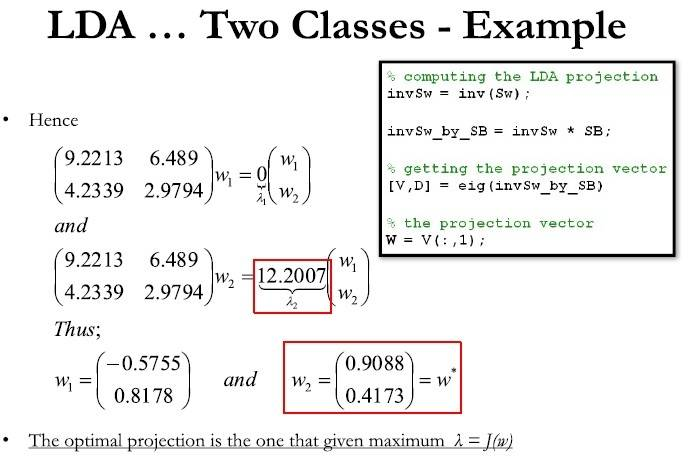# Solving equations for Eigenvector: Vanishing

## Homework Statement

I am trying to solve a Eigen vector matrix:

##\begin{bmatrix}9.2196& 6.488\\4.233& 2.9787\end{bmatrix}\cdot\begin{bmatrix}x\\y\end{bmatrix}-\lambda\begin{bmatrix}x\\y \end{bmatrix}=0##

I have found ##\lambda_1 = 0## and ##\lambda_2 = 12.1983##
However, I cant solve the following equations:
##9.2196x + 6.488y =0 -------(eq.1)##
and ##4.233x + 2.978y =0 ----(eq.2)##

I am multiplying ##(eq.1) ## by ##4.233x## and ##(eq.2)## by ##9.2196##

Some body please guide me how to solve these equations for ##x## and ##y## values.

Zulfi.

## Homework Equations

##9.2196x + 6.488y =0 -------(eq.1)##
and ##4.233x + 2.978y =0 ----(eq.2)##

## The Attempt at a Solution

I am multiplying ##(eq.1)## by ##4.233## and ##(eq.2)## by ##9.2196## but both the equations are vanishing. Some body please guide me.

Zulfi.

PeroK
Homework Helper
Gold Member
2021 Award

## Homework Statement

I am trying to solve a Eigen vector matrix:

##\begin{bmatrix}9.2196& 6.488\\4.233& 2.9787\end{bmatrix}\cdot\begin{bmatrix}x\\y\end{bmatrix}-\lambda\begin{bmatrix}x\\y \end{bmatrix}=0##

I have found ##\lambda_1 = 0## and ##\lambda_2 = 12.1983##
However, I cant solve the following equations:
##9.2196x + 6.488y =0 -------(eq.1)##
and ##4.233x + 2.978y =0 ----(eq.2)##

I am multiplying ##(eq.1) ## by ##4.233x## and ##(eq.2)## by ##9.2196##

Some body please guide me how to solve these equations for ##x## and ##y## values.

Zulfi.

## Homework Equations

##9.2196x + 6.488y =0 -------(eq.1)##
and ##4.233x + 2.978y =0 ----(eq.2)##

## The Attempt at a Solution

I am multiplying ##(eq.1)## by ##4.233## and ##(eq.2)## by ##9.2196## but both the equations are vanishing. Some body please guide me.

Zulfi.

The two equations are equivalent. So, you just take the solution of either one.

hilbert2
Gold Member
And, it only determines the solution up to a constant multiplier, so you can for example arbitrarily set ##x=1## and then find ##y##.

Hi,
Thanks for your response. Answer is different in the slide. I have attached the slide. Please guide me.#### Attachments

hilbert2
Gold Member
In the solution for ##w_1##, the ratio of the components ##\frac{0.8178}{0.5755}## is the same as the ratio ##\frac{9.2196}{6.488}## in your system of equations. So it's the same answer but with different normalization, one where ##x^2 + y^2 = 1##.

Hi,
##lambda_2 =12.1983##
I am not able to get the correct answer.

##9.2196x + 6.488y = 12.1983x ##
##9.216x - 12.1983x = -6.488y ##

##\begin{bmatrix}x\\y\end {bmatrix} = \begin{bmatrix}2.7\\1\end{bmatrix}##